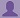SHARE
HELP

To start with, Sr2Jr’s first step is to reduce the expenses related to education. To achieve this goal Sr2Jr organized the textbook’s question and answers. Sr2Jr is community based and need your support to fill the question and answers. The question and answers posted will be available free of cost to all.Authors:
James Stewart
Chapter:
Functions And Models
Exercise:
Review
Question:1 | ISBN:9780538497909 | Edition: 7Question

(a) What is a function? What are its domain and range?
(b) What is the graph of a function?
(c) How can you tell whether a given curve is the graph of
a function?Answer

(a)   FUNCTION
We have 2 quantities (called "variables") and we observe there is a relationship between  them. If we find that for every value of the first variable there is only one value of the second variable, then we say:
"The second variable is a function of the first variable."
DOMAIN
The domain of a function is the complete set of possible values of the independent variable.\
RANGE
The range of a function is the complete set of all possible resulting values of the dependent variable (y, usually), after we have substituted the domain

(b) The Graph of a Function
The graph of a function is the set of all points whose co-ordinates (x, y) satisfy the function y= f{{\left({x}\right)}}y=f(x). This means that for each x-value there is a corresponding y-value which is obtained when we substitute into the expression for f{{\left({x}\right)}}f(x).
Since there is no limit to the possible number of points for the graph of the function, we will follow this procedure at first:
1. Select a few values of x (at least 5)
2.Obtain the corresponding values of the function and enter them into a table
3.Plot these points by joining them with a smooth curve

(c)   To test whether a graph of a curve is a function of x, one uses the vertical line test. To test whether a graph of a curve is a function of y, one uses the horizontal line test. If the function has an inverse, the graph of the inverse can be found by reflecting the graph of the original function over the line y = x.

Discussions

Post the discussion to improve the above solution.